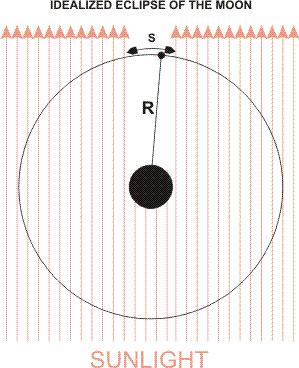# Experiment of The Month

## A Lunar Eclipse and the Distance to the MoonThe lunar eclipse scheduled for February 20, 2008 was the last fully visible in Pennsylvania until December 21, 2010. The eclipse began at 8:43 pm and continues until 12:09 am, with the Moon turning red during the totality phase of the eclipse (the red color is created by sunlight bending through the atmosphere, the same effect is seen at dawn and dusk making the Sun appear red).

The duration of the totality phase (when the Moon is red) can be used to estimate the distance from the Earth to the Moon. For the estimate described here, the important part is that you can do it yourself rather than the level of precision in the estimate.

The major assumption in the estimate is that the Earth's dark shadow (technically called the "umbra") is the same diameter as the Earth, when the shadow falls on the Moon to cause the eclipse. Then we know that when the edge of the Moon travels from the right side of the shadow to the left, it has traveled a distance equal to the diameter of the Earth.

In the figure the distance S is the distance along the Moon's orbit that lies in the Earth's shadow. In our approximation, S = (diameter of Earth) = 13,000km. (See Measuring Earth's Radius Along I-95 .) The ratio of S to the circumference of the Moon's orbit is
S/(2 p R)
where R is the radius of the Moon's orbit.

This ratio is the same as the ratio of the time spent in Earth's shadow to the time to complete one orbit.
(time for leading edge to cross shadow)/(28 days x 24hours/day) = S/(2 p R)

We can solve for R:

R=(13,000 km) x (28 days x 24hours/day)/(2px(time for leading edge to cross shadow))

This result is about twice as large as the measured distance to the Moon. The Sun is a disk, and its edges shine around the sides of the Earth, making the shadow smaller than in the drawing. The fully dark shadow of the Earth is only about half the Earth's diameter.

Still, the idea that you can measure the radius of the Earth for yourself, and estimate the distance to the Moon for yourself is important. Science is democratic in that way. Truth is what you can observe and deduce for yourself; not what an authority tells you.

## Experiment Of The Month

View past labs experiments.